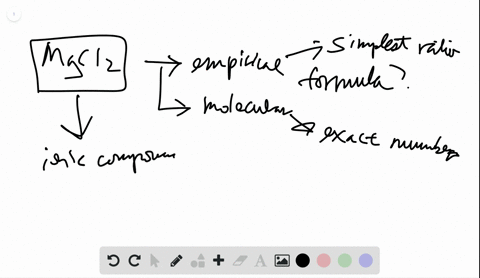🎉 The Study-to-Win Winning Ticket number has been announced! Go to your Tickets dashboard to see if you won! 🎉View Winning Ticket### Is $\mathrm{MgCl}_{2}$ an empirical or a molecula…

02:51University of Maine
Problem 34

# Which of the following sets of information allows you to obtain the molecular formula of a covalent compound? In each case that allows it, explain how you would proceed (draw a road map and write a Plan for a solution).(a) Number of moles of each type of atom in a given sample of the compound(b) Mass $\%$ of each element and the total number of atoms in a molecule of the compound(c) Mass $\%$ of each element and the number of atoms of one element in a molecule of the compound(d) Empirical formula and mass $\%$ of each element(e) Structural formula

## Discussion

You must be signed in to discuss.

## Video Transcript

to describe the composition or makeup of a chemical compound or molecule. There are three different ways in which we can describe the elements president. The first is called the empirical formula, which is the lowest whole number ratio of moles of each element in the compound. So for examples, a compound might have an empirical formula of C H three. This means for every mole of carbon, there are three moles of hydrogen. The molecular formula is the actual ratio of moles of Utah, element in a compound, and it's usually a multiple of the empirical formula. So a molecular formula for a compound that exists to see to H six and you can see that this means that there are two moles of carbon and six moles of hydrogen in one mole of the compound, you can see this is a multiple of the empirical formula. Finally, a structural formula is a picture or a drawing that shows how everything is arranged in the Adam. So this is usually the molecular formula, but drawn as a picture. So this would be an example of a structural formula. You have two carbons and six hydrogen around each carbon, so the question is, what information do you need to have to determine the molecular formula? If you know the number of moles of each type of Adam and the sample of compound, this is Onley enough to tell you the empirical formula. So a is not enough information. If you have the mass of each element and the total number of atoms in a molecule the compound you can use this information to find the molecular formula. The first step is ticked, a mass of each element and change it to moles. And you do this by using the molar mass. Once you have the moles of each element, you confined the ratio. And this allows you to write the empirical formula. To find the molecular formula, we have to compare total number of Adams and the empirical formula to the total number of atoms in the molecule. So after you found the empirical formula, you find total number of atoms in the molecule divided by the atoms. In the empirical formula. This gives us the multiplier and then you can find the molecular formula. Similarly, if you have the mass, each element in the total number of Adams of only one element. We can still use that to find the ratio of the empirical formula to molecular formula. So see also gives us enough information. Follow the same steps to find the empirical formula. And then we confined the ratio between the number of atoms of one element in the empirical formula and the Adams one element in a molecule, and this again gives us the multiplier to find the molecular formula. If we know the empirical formula and massive each element, that is not enough information to find the ratio between molecular an empirical formula. Finally, given a structural formula, this is perhaps the most informative, and you can use it to find the molecular formula, and you do this simply by counting up the number of each Adam present.